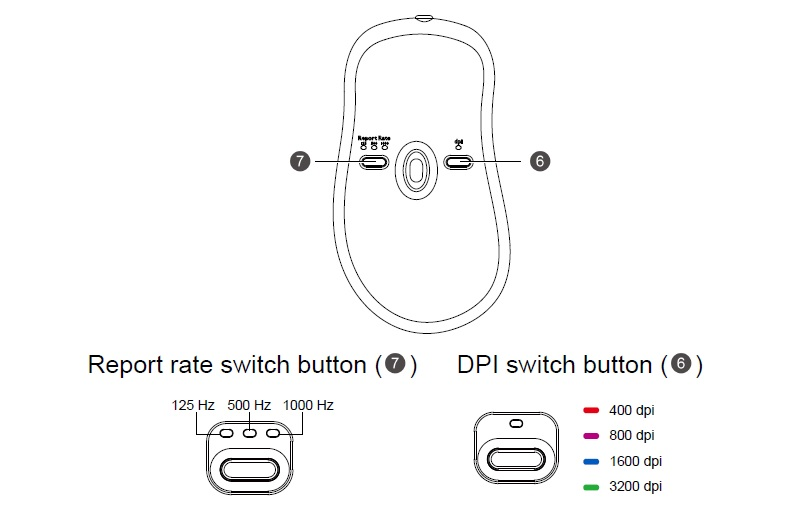# 該如何調整ZOWIE滑鼠的DPI與Polling Rate ?#### 適用產品型號

EC1-B (L), EC2-B (M), EC1-B CS:GO (L), EC2-B CS:GO (M), EC1 TYLOO (L), EC2 TYLOO (M), EC1 (L), EC2 (M), EC1-B DIVINA BLUE (L), EC1-B DIVINA PINK (L), EC2-B DIVINA BLUE (M), EC2-B DIVINA PINK (M), FK1-B (L), FK2-B (M), FK1+-B (XL), FK1-B DIVINA BLUE (L), FK1-B DIVINA PINK (L), FK2-B DIVINA BLUE (M), FK2-B DIVINA PINK (M), FK1+-B DIVINA BLUE (XL), FK1+-B DIVINA PINK (XL), ZA11-B (L), ZA12-B (M), ZA13-B (S), S1 (M), S2 (S), S1 DIVINA BLUE (M), S1 DIVINA PINK (M), S2 DIVINA BLUE (S), S2 DIVINA PINK (S), ZA11-B (L), ZA12-B (M), ZA13-B (S), EC1-C (L), EC2-C (M), EC3-C (S), FK1+-C (XL), FK1-C (L), FK2-C (M), EC1-C (L), EC2-C (M), EC3-C (S), FK1+-C (XL), FK1-C (L), FK2-C (M), S1-C (M), S2-C (S), ZA11-C (L), ZA12-C (M), ZA13-C (S)